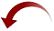# Time Zones, Windows, and Microsoft Office - Part 2

Published on
3,322 Points
122 Views
2 Endorsements
Listing and selecting time zones in Microsoft Access and Excel is not straight forward. In the previous article was shown how to retrieve the time zones of Windows. Here will be demonstrated how to create tables to store these and how to display and select a time zone in Microsoft Access and Excel.

This is the second article about maximising the usage of time zones in Windows with Microsoft Access and Excel.
The first covers the VBA code to retrieve the time zones:
Time Zones, Windows, and VBA - Part 1.

## Presenting the time zones and their bias in Microsoft Access

The last topic in the first article was how to format the bias of a time zone, and the function FormatBias for the purpose was discussed. With this tool - to format the bias for display - it is time to present the time zones and their bias or other information to the user.

A ComboBox or ListBox is well suited for the purpose and - as the list isn't that large and we have no table to use as a row source - a callback function is the perfect choice for filling the ComboBox or ListBox.
How to create a callback function is a lecture on its own, so browse for a guide, if you are not familiar with this (often labelled) hidden gem of Access. Read the guide and peruse the example code here. It will pay off.

The callback function here will list the time zones and their MUI:

```' Common constants.

' Default column width.
Private Const DefaultColumnWidth    As Integer = -1
' Hidden column width.
Private Const HiddenColumnWidth     As Integer = 0
'

' Callback function to list the timezones of Windows.
'
' Example for retrieval of selected value:
'
'   Dim TimezoneName    As String
'   TimezoneName = Me!ControlName.Value
'
' Typical settings for combobox or listbox:
'
'   ControlSource:  Bound or unbound
'   RowSource:      Leave empty
'   RowSourceType:  CallWindowsTimezones
'   BoundColumn:    1
'   LimitToList:    Yes
'   AllowEditing:   No
'   Format:         A valid format for date values
'   ColumnCount:    Don't care. Will be set by the function
'   ColumnWidths:   Don't care. Will be overridden by the function
'
' 2019-12-12. Cactus Data ApS, CPH.
'
Public Function CallWindowsTimezones( _
Control As Control, _
Id As Long, _
Row As Long, _
Column As Long, _
Code As Integer) _
As Variant

' Fixed constants.
'
' Count of columns in the control.
'   0: Key. Name of the timezone.
'   1: Mui.
'   2: Custom display.
Const ColumnCount   As Integer = 3

' Customisable constants.
'
' Separator for use in Headers.
Const Separator     As String = ";"
' Count of items in Headers must match ColumnCount.
Const Headers       As String = "Name" & Separator & "Mui" & Separator & "Timezone offset and locations"

Static ColumnWidth(0 To ColumnCount - 1) As Integer
Static RowCount     As Integer
Static Entries()    As TimezoneEntry

Dim Entry           As TimezoneEntry
Dim Value           As Variant

Select Case Code
Case acLBInitialize
' Control settings.
Control.ColumnCount = ColumnCount   ' Set the column count of the control.
ColumnWidth(0) = HiddenColumnWidth  ' Hide the bound (value) column.
ColumnWidth(1) = HiddenColumnWidth  ' Hide the Mui column.
ColumnWidth(2) = DefaultColumnWidth ' Set the width of the display column to the default width.

' Value settings.
If RowCount > 0 Then
' Entries has been retrieved.
Else
Entries = RegistryTimezoneItems()
SortEntriesBiasLocations Entries
' Count of rows to display including column headings.
RowCount = 1 + UBound(Entries) + Abs(Control.ColumnHeads)
End If

' Initialize.
Value = True                        ' True to initialize.
Case acLBOpen
Value = Timer                       ' Autogenerated unique ID.
Case acLBGetRowCount                    ' Get count of rows.
Value = RowCount                    ' Set count of rows.
Case acLBGetColumnCount                 ' Get count of columns.
Value = ColumnCount                 ' Set count of columns.
Case acLBGetColumnWidth                 ' Get the column width.
Value = ColumnWidth(Column)         ' Use preset column widths.
Case acLBGetValue                       ' Get the data for each row and column.
If Control.ColumnHeads = True And Row = 0 Then
Else
' Display a value.
Select Case Column
Case 0
Value = Entry.Name
Case 1
Value = Entry.Mui
Case 2
Value = FormatBias(Entry.Bias, True, True, Entry.Name) & " " & Entry.Locations
End Select
End If
Case acLBGetFormat                      ' Format the data.
' Use default (standard) format.    ' Apply the value or display format.
Case acLBClose                          ' The form closes or the control is requeried.
' no-op.
Case acLBEnd                            ' The form closes or the control is requeried.
' no-op.
End Select

' Return Value.
CallWindowsTimezones = Value

End Function```
It is used in the form that is included in the attached demo.

The implementation is extremely simple. In the properties of the ComboBox, set the RowSourceType to the name of the function: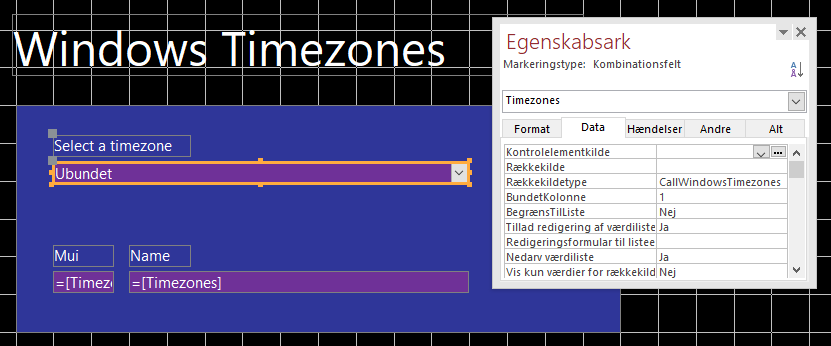When launched, the function will itself set the other crucial properties of the ComboBox (see section acLBInitialize in the code above).

Now, open the form, click dropdown to make a selection, and you will see the equivalence to the list of Windows 10: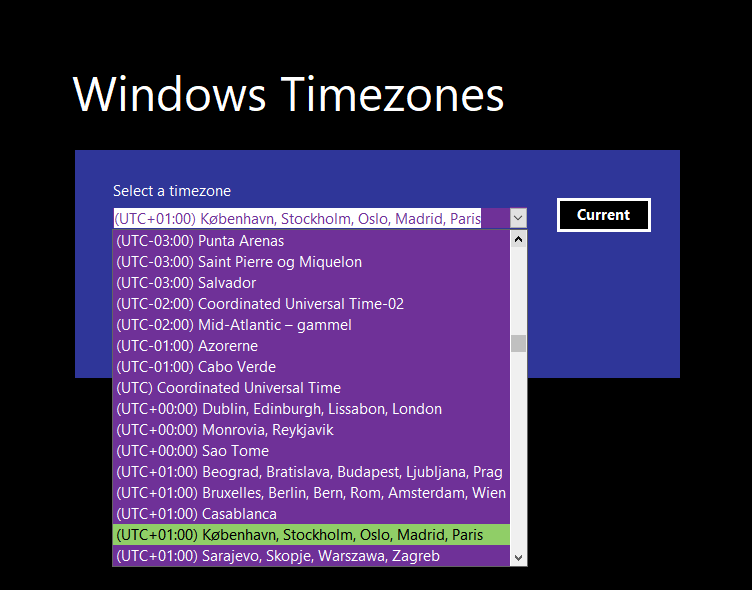and - having made a selection - the MUI and the Name (which you may recall is the true key) of the selected time zone are displayed: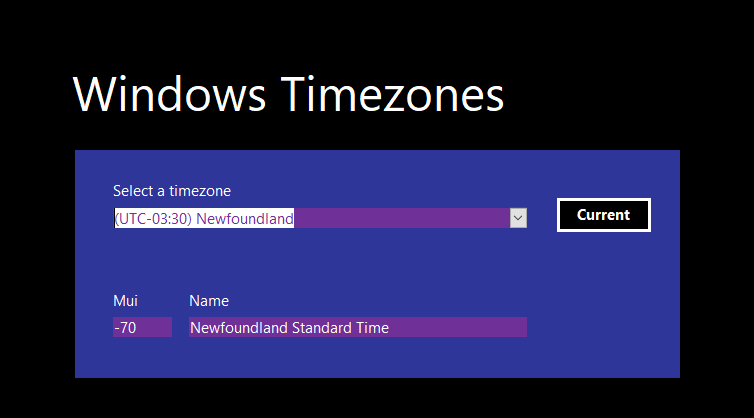You may wonder, why all MUIs are a negative number, but no documentation can be found that explains or just indicates the reason. It has just happened to be so.

Of course, the callback function can be modified or expanded to return any other information about the time zones.

## Tables for extensive calls, sorting, filtering, and searching

If time zones and their data are expected to be heavily used in an application, it will be more convenient to normalise them and keep them in two tables where the child table holds the locations. Then the data can be accessed in a flexible way in queries and code like other data kept in tables.

There will be no fixed definition for the table structure, but included in the demo application is code that will generate and fill a pair of local tables that will fit most purposes. As it is unique, the MUI has been chosen to be the key, not the name of the time zone:

```' Timezone table names.
Private Const TimezoneTableZone     As String = "WindowsTimezone"
Private Const TimezoneTableLocation As String = "WindowsTimezoneLocation"
Private Const TimezoneTableRelation As String = TimezoneTableZone & "_" & TimezoneTableLocation```
Pure VBA is used to create and fill the tables. Call the function CreateTimezoneData and, in a snap, you will have the tables ready for use:

```' Creates (if missing) the supporting timezone tables.
' Returns True if success, False if not.
'
' 2018-11-01. Gustav Brock. Cactus Data ApS, CPH.
'
Public Function CreateTimezoneData() As Boolean

Dim Result  As Boolean

' Create the timezone tables if missing.
Result = CreateTimezoneDataTable(TimezoneTableZone)
Result = Result And CreateTimezoneDataTable(TimezoneTableLocation)

If Result = True Then
' Enforce referential integrity on the timezone tables.
Result = CreateTimezoneDataTableRelations()
End If

CreateTimezoneData = Result

End Function```
The function calls a helper function, CreateTimezoneDataTable, twice to create the two tables complete with indexes:

```' Creates a timezone table and its indexes from scratch if missing.
' Returns True if success, False if not.
'
' 2018-11-01. Gustav Brock. Cactus Data ApS, CPH.
'
Public Function CreateTimezoneDataTable( _
ByVal TableName As String) _
As Boolean

Const PrimaryKeyName    As String = "PrimaryKey"

Dim Database            As DAO.Database
Dim Table               As DAO.TableDef
Dim Field               As DAO.Field
Dim Index               As DAO.Index

Dim Result              As Boolean

Set Database = CurrentDb

If IsTableDefName(TableName) Then
Result = True
Else
' Create table.
Select Case TableName
Case TimezoneTableZone
Set Table = Database.CreateTableDef(TableName)
Set Field = Table.CreateField(TimezoneMui, dbInteger)
Field.Required = True
Table.Fields.Append Field
Set Field = Table.CreateField(TimezoneMuiDaylight, dbInteger)
Field.Required = True
Table.Fields.Append Field
Set Field = Table.CreateField(TimezoneMuiStandard, dbInteger)
Field.Required = True
Table.Fields.Append Field
Set Field = Table.CreateField(TimezoneName, dbText, 50)
Field.AllowZeroLength = False
Field.Required = True
Table.Fields.Append Field
Set Field = Table.CreateField(TimezoneBias, dbInteger)
Field.Required = True
Table.Fields.Append Field
Set Field = Table.CreateField(TimezoneUtc, dbText, 50)
Field.AllowZeroLength = False
Field.Required = True
Table.Fields.Append Field
Set Field = Table.CreateField(TimezoneLocations, dbText, 50)
Field.AllowZeroLength = False
Field.Required = True
Table.Fields.Append Field
Set Field = Table.CreateField(TimezoneDlt, dbText, 50)
Field.AllowZeroLength = False
Field.Required = True
Table.Fields.Append Field
Set Field = Table.CreateField(TimezoneStd, dbText, 50)
Field.AllowZeroLength = False
Field.Required = True
Table.Fields.Append Field
Set Field = Table.CreateField(TimezoneFirstEntry, dbInteger)
Field.Required = False
Field.DefaultValue = "Null"
Table.Fields.Append Field
Set Field = Table.CreateField(TimezoneLastEntry, dbInteger)
Field.Required = False
Field.DefaultValue = "Null"
Table.Fields.Append Field

Set Index = Table.CreateIndex(PrimaryKeyName)
Set Field = Index.CreateField(TimezoneMui)
Index.Fields.Append Field
Index.Primary = True
Table.Indexes.Append Index
Set Index = Table.CreateIndex(TimezoneMuiDaylight)
Set Field = Index.CreateField(TimezoneMuiDaylight)
Index.Fields.Append Field
Index.Unique = True
Index.Primary = False
Table.Indexes.Append Index
Set Index = Table.CreateIndex(TimezoneMuiStandard)
Set Field = Index.CreateField(TimezoneMuiStandard)
Index.Fields.Append Field
Index.Unique = True
Index.Primary = False
Table.Indexes.Append Index
Set Index = Table.CreateIndex(TimezoneName)
Set Field = Index.CreateField(TimezoneName)
Index.Fields.Append Field
Index.Unique = True
Index.Primary = False
Table.Indexes.Append Index

Case TimezoneTableLocation
Set Table = Database.CreateTableDef(TableName)
Set Field = Table.CreateField(TimezoneLocationId, dbLong)
Field.Required = True
Field.Attributes = Field.Attributes Or dbAutoIncrField
Table.Fields.Append Field
Set Field = Table.CreateField(TimezoneLocationMui, dbInteger)
Field.Required = True
Table.Fields.Append Field
Set Field = Table.CreateField(TimezoneLocationName, dbText, 50)
Field.AllowZeroLength = False
Field.Required = True
Table.Fields.Append Field

' Don't create an index on MUI as this will
' be created when creating referential integrity.
Set Index = Table.CreateIndex(PrimaryKeyName)
Set Field = Index.CreateField(TimezoneLocationId)
Index.Fields.Append Field
Index.Primary = True
Table.Indexes.Append Index
Set Index = Table.CreateIndex(TimezoneLocationName)
Set Field = Index.CreateField(TimezoneLocationName)
Index.Fields.Append Field
Table.Indexes.Append Index

End Select

If Not Table Is Nothing Then
' Append table.
Database.TableDefs.Append Table
Result = True
End If
End If

CreateTimezoneDataTable = Result

End Function```
and then a function, CreateTimezoneDataTableRelations, to create the relation between the two tables:

```' Creates and appends missing relations between the timezone tables.
' Note, that this will create a hidden index on the foreign table field.
' Returns True if success, False if not, typically because the tables are missing.
'
' 2018-11-01. Gustav Brock. Cactus Data ApS, CPH.
'
Public Function CreateTimezoneDataTableRelations() As Boolean

Dim Database        As DAO.Database
Dim Field           As DAO.Field
Dim Relation        As DAO.Relation
Dim Table           As DAO.TableDef
Dim ForeignTable    As DAO.TableDef

Dim Name            As String
Dim ForeignName     As String
Dim Result          As Boolean

Set Database = CurrentDb

If IsRelationName(TimezoneTableRelation) Then
Result = True
ElseIf IsTableDefName(TimezoneTableZone) And IsTableDefName(TimezoneTableLocation) Then
Set Table = Database.TableDefs(TimezoneTableZone)
Set ForeignTable = Database.TableDefs(TimezoneTableLocation)

' Create and append relation RelationName using these fields:
Name = TimezoneMui
ForeignName = TimezoneLocationMui

Set Relation = Database.CreateRelation(TimezoneTableRelation)
Relation.Table = Table.Name
Relation.ForeignTable = ForeignTable.Name

Set Field = Relation.CreateField(Name)
Field.ForeignName = ForeignName
Relation.Fields.Append Field
Database.Relations.Append Relation

Set ForeignTable = Nothing
Set Table = Nothing
Result = True
End If

CreateTimezoneDataTableRelations = Result

End Function```
Finally, the function ReloadWindowsTimezoneTables will fill or reload the two tables - and even create them should they not exist - in one go:

```' Updates the local timezone tables with the current timezones of Windows.
' If Force is True, the tables will be created if they don't exist.
' Returns True if the tables were created or updated successfully.
'
' 2018-11-12. Gustav Brock. Cactus Data ApS, CPH.
'
Optional ByVal Force As Boolean) _
As Boolean

Dim Timezones   As DAO.Recordset
Dim Locations   As DAO.Recordset

Dim Entries()   As TimezoneEntry
Dim Items()     As String

Dim Index       As Integer
Dim SubIndex    As Integer
Dim Sql         As String
Dim Success     As Boolean

If Force = True Then
' Create the timezone tables if they don't exist.
Success = CreateTimezoneData()
Else
' Check if the timezone tables exist.
Success = IsRelationName(TimezoneTableZone)
End If

If Success Then
Entries = RegistryTimezoneItems()

Sql = "Delete * From " & TimezoneTableLocation & ""
CurrentDb.Execute Sql
Sql = "Delete * From " & TimezoneTableZone & ""
CurrentDb.Execute Sql

Sql = "Select * From " & TimezoneTableZone & ""
Set Timezones = CurrentDb.OpenRecordset(Sql)
Sql = "Select * From " & TimezoneTableLocation & ""
Set Locations = CurrentDb.OpenRecordset(Sql)

For Index = LBound(Entries) To UBound(Entries)
Timezones.Fields(TimezoneMui).Value = Entries(Index).Mui
Timezones.Fields(TimezoneMuiDaylight).Value = Entries(Index).MuiDaylight
Timezones.Fields(TimezoneMuiStandard).Value = Entries(Index).MuiStandard
Timezones.Fields(TimezoneName).Value = Entries(Index).Name
Timezones.Fields(TimezoneBias).Value = Entries(Index).Bias
Timezones.Fields(TimezoneUtc).Value = Entries(Index).Utc
Timezones.Fields(TimezoneLocations).Value = Entries(Index).Locations
Timezones.Fields(TimezoneDlt).Value = Entries(Index).ZoneDaylight
Timezones.Fields(TimezoneStd).Value = Entries(Index).ZoneStandard
Timezones.Fields(TimezoneFirstEntry).Value = Entries(Index).FirstEntry
Timezones.Fields(TimezoneLastEntry).Value = Entries(Index).LastEntry
Timezones.Update

Items = Split(Entries(Index).Locations, ",")
For SubIndex = LBound(Items) To UBound(Items)
If Trim(Items(SubIndex)) <> "" Then
Locations.Fields(TimezoneLocationMui).Value = Entries(Index).Mui
Locations.Fields(TimezoneLocationName).Value = Trim(Items(SubIndex))
Locations.Update
End If
Next
Next
Locations.Close
Timezones.Close
Success = True
End If

End Function```
The full process is quite speedy - about half a second.

You can now conveniently browse the tables with the time zones and their locations: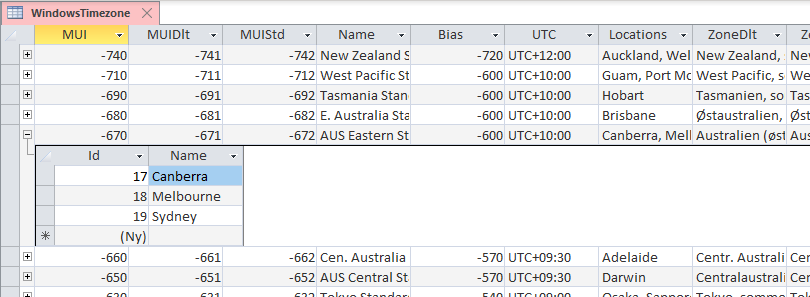Having these tables as a source, all sorts of filtering, querying, and lookups will become trivial.

Do note, that if you wish to find the Daylight Saving Time (DST) bias for a date, you can check the values for FirstEntry and LastEntry to see if DST is applied for the time zone and, if so, in which range of years the parameters for DST have been changed:

• If FirstEntry is Null or after the year you wish to look up, DST was not introduced
• If the year to look up falls between FirstEntry and LastEntry, you must look up the bias specifically for the year
• If LastEntry is Null or earlier than the year you wish to look up, the current bias is the value to use

## Presenting the time zones and their bias in Microsoft Excel

In Excel, something similar to what we did above in Access can be achieved as well, though in a very different way: A combination of formulas, code, and ListObject tables can be used to replace the callback function and the data tables.

#### Worksheet with tables

The first step is to create a worksheet holding the data, and ListObject tables are ideal for the purpose.

As above for Access, this can be done completely from code rather than creating the tables manually. I can strongly recommend this method, as it is a huge time saver in case you wish to modify the data worksheet and table structure.

First, we need a set of constants to not mess up the code:

```' Timezone worksheet name.
Private Const TimezoneWorksheetName As String = "Data"

' Timezone table names.
Private Const TimezoneTableZone     As String = "WindowsTimezone"
Private Const TimezoneTableLocation As String = "WindowsTimezoneLocation"

' Timezone table positions.
Private Const TimezoneRowIndex      As Integer = 1
Private Const TimezoneIndex         As Integer = 1
Private Const TimezoneLocationIndex As Integer = 14

' Timezone field names.
' A field for the Registry key TZI is not included.
Private Const TimezoneMui           As String = "MUI"
Private Const TimezoneMuiDaylight   As String = "MUIDlt"
Private Const TimezoneMuiStandard   As String = "MUIStd"
Private Const TimezoneBias          As String = "Bias"
Private Const TimezoneName          As String = "Name"
Private Const TimezoneUtc           As String = "UTC"
Private Const TimezoneLocations     As String = "Locations"
Private Const TimezoneDlt           As String = "ZoneDlt"
Private Const TimezoneStd           As String = "ZoneStd"
Private Const TimezoneFirstEntry    As String = "FirstEntry"
Private Const TimezoneLastEntry     As String = "LastEntry"
Private Const TimezoneDisplay       As String = "Display"
Private Const TimezoneLocationId    As String = "Id"
Private Const TimezoneLocationMui   As String = "MUI"
Private Const TimezoneLocationName  As String = "Name"```
Then, create and return the Worksheet to hold the tables:

```' Look up and return the worksheet holding the timezone tables.
'
' 2020-03-01. Gustav Brock. Cactus Data ApS, CPH.
'
Public Function WorksheetData() As Excel.Worksheet

Dim Worksheet       As Excel.Worksheet

Dim Index           As Integer

On Error GoTo WorksheetData_Error

Index = WorksheetIndex(ThisWorkbook, TimezoneWorksheetName)
If Index = 0 Then
Worksheet.Name = TimezoneWorksheetName
RenameWorksheetModule ThisWorkbook, Worksheet.Index, TimezoneWorksheetName
Else
Set Worksheet = ThisWorkbook.Worksheets(Index)
End If

Set WorksheetData = Worksheet

WorksheetData_Exit:
Exit Function

WorksheetData_Error:
MsgBox "Error " & Err.Number & " (" & Err.Description & ") in procedure WxslData.WorksheetData."
Resume WorksheetData_Exit

End Function```
This function is used in the top-level function to create and fill the tables:

```' Fill the timezone tables from the Windows Registry.
' They will be ordered by their bias and list of localised locations.
' Returns True, if the tables were successfully filled.
'
' If the worksheet does not exist, it will be created.
' If the tables don't exist, they will be create of no other tables are present.
'
' 2020-03-01. Gustav Brock. Cactus Data ApS, CPH.
'

Dim Worksheet       As Excel.Worksheet
Dim TimezoneList    As Excel.ListObject
Dim LocationList    As Excel.ListObject
Dim ListColumn      As Excel.ListColumn
Dim Range           As Excel.Range

Dim Entries()       As TimezoneEntry
Dim Items()         As String

Dim Entry           As TimezoneEntry
Dim Index           As Integer
Dim SubIndex        As Integer
Dim Id              As Integer
Dim Result          As Boolean

Entries = RegistryTimezoneItems()
SortEntriesBiasLocations Entries

Set Worksheet = WorksheetData
If Not Worksheet Is Nothing Then
If Worksheet.ListObjects.Count = 0 Then
' Worksheet exists but has no tables.
' Create the tables.
CreateTimezoneData
Else
' Do not overwrite existing tables.
End If

If IsListObject(Worksheet, TimezoneTableZone) And IsListObject(Worksheet, TimezoneTableLocation) Then
' Tables exist in the worksheet.
' Clear their content if they priviously have been filled.
Set LocationList = Worksheet.ListObjects(TimezoneTableLocation)
If Not LocationList.DataBodyRange Is Nothing Then
LocationList.DataBodyRange.Delete
End If
Set TimezoneList = Worksheet.ListObjects(TimezoneTableZone)
If Not TimezoneList.DataBodyRange Is Nothing Then
TimezoneList.DataBodyRange.Delete
End If

' Fill the tables row by row..
For Index = LBound(Entries) To UBound(Entries)
' Add and fill a row for a timezone.
Entry = Entries(Index)
Range(1, 1) = Entry.Mui
Range(1, 2) = Entry.MuiDaylight
Range(1, 3) = Entry.MuiStandard
Range(1, 4) = Entry.Name
Range(1, 5) = Entry.Bias
Range(1, 6) = Entry.Utc
Range(1, 7) = Entry.Locations
Range(1, 8) = Entry.ZoneDaylight
Range(1, 9) = Entry.ZoneStandard
Range(1, 10) = Entry.FirstEntry
Range(1, 11) = Entry.LastEntry
' The formatted column for display and validation.
Range(1, 12) = FormatBias(Entry.Bias, True, True, Entry.Name) & " " & Entry.Locations

' Add and fill rows for the locations of the timezone.
Items = Split(Entry.Locations, ",")
For SubIndex = LBound(Items) To UBound(Items)
If Trim(Items(SubIndex)) <> "" Then
Id = Id + 1
Range(1, 1) = Id
Range(1, 2) = Entry.Mui
Range(1, 3) = Trim(Items(SubIndex))
End If
Next
Next
Result = True

' Adjust column widths for both tables to fit the content.
For Index = 1 To TimezoneList.ListColumns.Count
Set ListColumn = TimezoneList.ListColumns(Index)
ListColumn.Range.EntireColumn.AutoFit
Next
For Index = 1 To LocationList.ListColumns.Count
Set ListColumn = LocationList.ListColumns(Index)
ListColumn.Range.EntireColumn.AutoFit
Next
Else
' No tables (ListObjects) to fill.
End If
End If

Exit Function

MsgBox "Error " & Err.Number & " (" & Err.Description & ") in procedure WxlsData.ReloadTimezones."

End Function```
Inspecting the function, you'll see that after having verified/created the worksheet, the tables are created by the function CreateTimezoneData, which calls the function CreateTimezoneDataTable twice to create the tables one by one:

```' Create and prepare a worksheet to hold the tables for the timezones.
' Returns True if the worksheet and tables existed or were created.
'
' 2020-03-01. Gustav Brock. Cactus Data ApS, CPH.
'
Public Function CreateTimezoneData() As Boolean

Dim Worksheet       As Excel.Worksheet

Dim Result          As Boolean

' Fetch or create the worksheet holding the tables.
Set Worksheet = WorksheetData

If Not Worksheet Is Nothing Then
' Check that the tables are present. If not, they will be created.
Result = CreateTimezoneDataTable(Worksheet, TimezoneTableZone)
If Result = True Then
Result = CreateTimezoneDataTable(Worksheet, TimezoneTableLocation)
End If
End If

CreateTimezoneData = Result

End Function

' Create and prepare a table for the timezones.
' Returns True if the table existed or were created.
'
' 2020-03-01. Gustav Brock. Cactus Data ApS, CPH.
'
Public Function CreateTimezoneDataTable( _
ByRef Worksheet As Excel.Worksheet, _
ByVal TableName As String) _
As Boolean

Dim ListObject              As Excel.ListObject
Dim Range                   As Excel.Range
Dim ListColumn              As Excel.ListColumn

Dim ColumnNames             As Variant
Dim ColumnIndex             As Integer
Dim Result                  As Boolean

On Error GoTo CreateTimezoneDataTable_Error

Select Case TableName
Case TimezoneTableZone
Set Range = Worksheet.Cells(TimezoneRowIndex, TimezoneIndex)
Case TimezoneTableLocation
Set Range = Worksheet.Cells(TimezoneRowIndex, TimezoneLocationIndex)
End Select

If Not IsListObject(Worksheet, TableName) Then
' Create the table.
Set ListObject = Worksheet.ListObjects.Add(xlSrcRange, Range, , xlYes)
ListObject.Name = TableName

Select Case TableName
Case TimezoneTableZone
ColumnNames = Array( _
TimezoneMui, _
TimezoneMuiDaylight, _
TimezoneMuiStandard, _
TimezoneName, _
TimezoneBias, _
TimezoneUtc, _
TimezoneLocations, _
TimezoneDlt, _
TimezoneStd, _
TimezoneFirstEntry, _
TimezoneLastEntry, _
TimezoneDisplay)

Case TimezoneTableLocation
ColumnNames = Array( _
TimezoneLocationId, _
TimezoneLocationMui, _
TimezoneLocationName)
End Select

For ColumnIndex = LBound(ColumnNames) + 1 To UBound(ColumnNames)
Next
For ColumnIndex = LBound(ColumnNames) To UBound(ColumnNames)
Set ListColumn = ListObject.ListColumns(ColumnIndex + 1)
ListColumn.Name = ColumnNames(ColumnIndex)
ListColumn.Range.EntireColumn.AutoFit
Next
' The table was created.
Result = True
Else
' The table is present.
Result = True
End If

CreateTimezoneDataTable = Result

CreateTimezoneDataTable_Exit:
Exit Function

CreateTimezoneDataTable_Error:
MsgBox "Error " & Err.Number & " (" & Err.Description & ") in procedure WxlsData.CreateTimezoneDataTable."
Resume CreateTimezoneDataTable_Exit

End Function```
After having verified that the tables have been created using the helper function IsListObject (found in module Common and not listed here as is does exactly as it is named), the function clears the tables and populates them from the array Entries filled in the first code line of the function.

The first table holding the time zones will appear similar to this using the localisation of your Excel installation: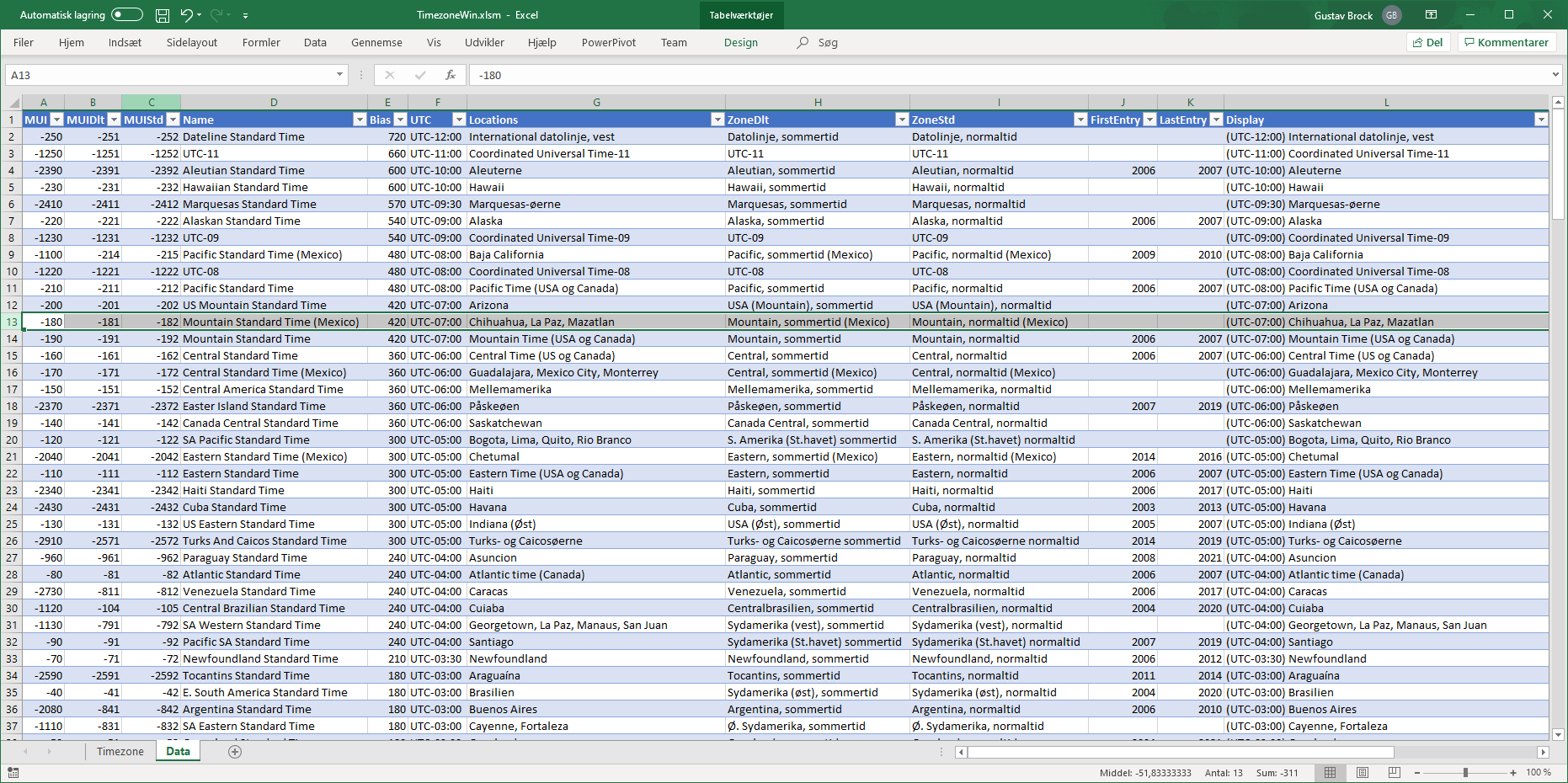The other table holding the locations has only a few columns: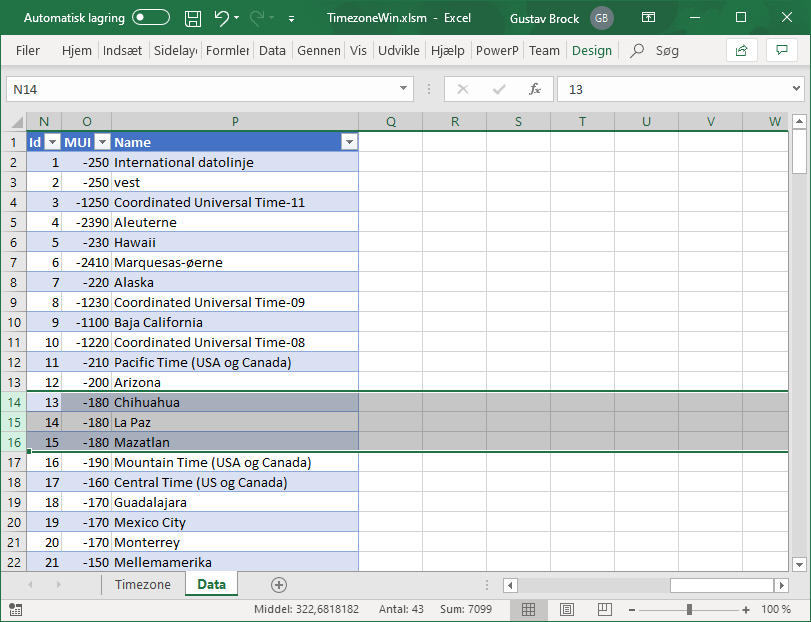#### Worksheet for presentation

Next step to prepare for the presentation of the time zones, is to create a collection of Named Ranges in addition to those already created for the tables: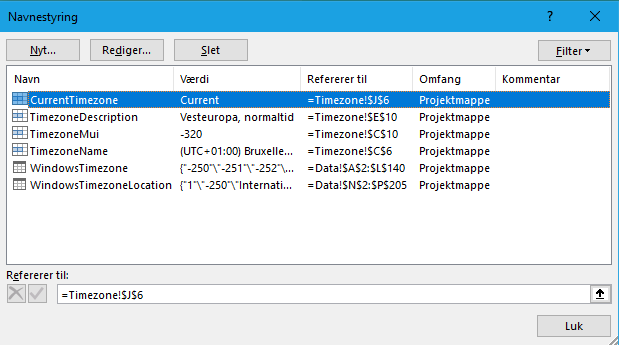The first, CurrentTimezone, is for a "pseudo button" which - when selected - will retrieve the current time zone for display.
The two next are for the cells that will display information for the time zone selected by the value entered in the cell which TimezoneName refers to.

To make that cell appear as a ComboBox, data validation is applied:

• Select the timezone cell
• Select "Data Validation" from the band
• First tab: Select "List" and set mark for displaying a ComboBox (the red box)
• Input the formula for where to look up the values (the Display column in the table):

`=INDIRECT("WindowsTimezone[Display]")`
• Last tab: Adjust type, title, and message as to your liking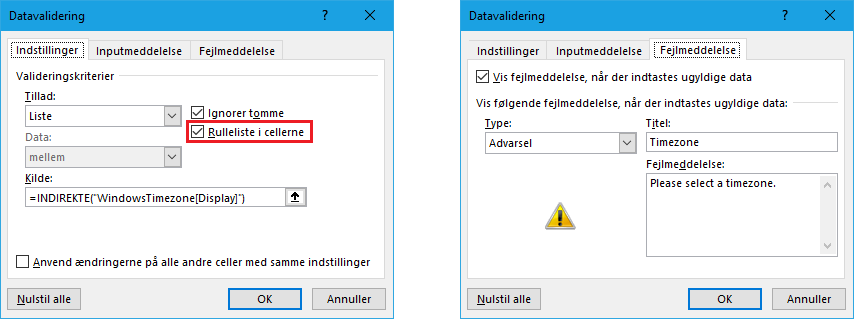When ready, create formulas for the two display cells, TimezoneMUI and TimezoneDescription respectively:

```=INDEX(WindowsTimezone[MUI];MATCH(TimezoneName;WindowsTimezone[Display];0))
=INDEX(WindowsTimezone[ZoneStd];MATCH(TimezoneName;WindowsTimezone[Display];0))```
Next step is to transform the "pseudo button" into doing something when selected. A tiny piece of code is needed for this:

```' Have a cell select to simulate a button click.
'
Private Sub Worksheet_SelectionChange(ByVal Target As Range)

' Named Range used as "button".
Const ButtonName            As String = "CurrentTimezone"
' Named Range holding the timezone name (the key).
Const TimezoneName          As String = "TimezoneName"

Dim ButtonRange             As Excel.Range
Dim NameRange               As Excel.Range

Set ButtonRange = ThisWorkbook.Names(ButtonName).RefersToRange
Set NameRange = ThisWorkbook.Names(TimezoneName).RefersToRange

' The user has selected the "button" and only this.
' Set the timezone to the current timezone displayed in "Windows style".
NameRange.Value = FormatBias(TimezoneCurrent.Bias, True, True, TimezoneCurrent.Name) & " " & TimezoneCurrent.Locations

' Reset formulas if needed.
ResetReferences
End If

End Sub```
The in-line comments explain the steps. The last step is to reset the formulas for the two look up cells, as these lose their references if the worksheet with the tables is not present:

```' Reset the formulas for the timezone look up cells
' if the references for these have been lost.
'
Private Sub ResetReferences()

Const MuiName               As String = "TimezoneMui"
Const DescriptionName       As String = "TimezoneDescription"

Const MuiFormula            As String = "=INDEX(WindowsTimezone[MUI],MATCH(TimezoneName,WindowsTimezone[Display],0))"
Const DescriptionFormula    As String = "=INDEX(WindowsTimezone[ZoneStd],MATCH(TimezoneName,WindowsTimezone[Display],0))"

Dim MuiRange                As Excel.Range
Dim DescriptionRange        As Excel.Range

Set MuiRange = ThisWorkbook.Names(MuiName).RefersToRange
Set DescriptionRange = ThisWorkbook.Names(DescriptionName).RefersToRange

If MuiRange.Formula <> MuiFormula Then
MuiRange.Formula = MuiFormula
End If
If DescriptionRange.Formula <> DescriptionFormula Then
DescriptionRange.Formula = DescriptionFormula
End If

End Sub```
Finally, style the worksheet with colours and fonts as to your preferences. This is from the attached demo workbook: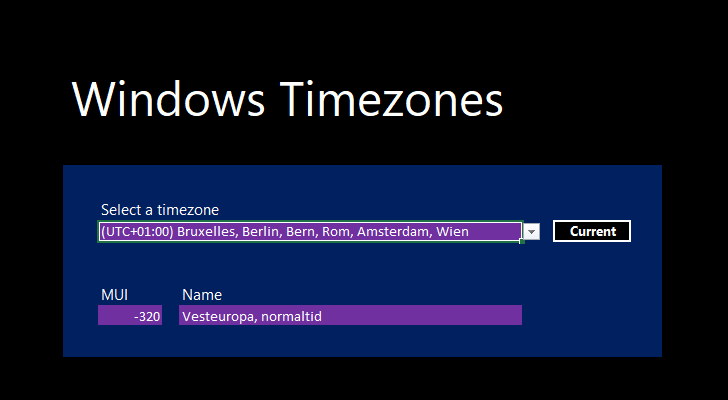and with the dropdown activated - having the dull design of Excel you cannot control: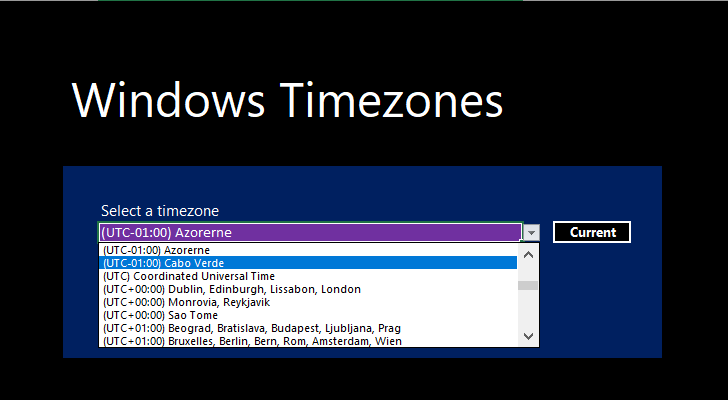## Conclusion

Having taken advantage of the collection of functions from the first article, it has been demonstrated how to store and display information in Microsoft Access and Excel about time zones retrieved from the Windows Registry without any third-party tools or need for supplemental information.
Also, some methods for optimising the user interface to meet the expectations of a demanding user has been shown.
Finally, sample applications ready to use have been attached for those who wish at once to be familiar with the concepts or to be used as a skeleton for parts of other applications.

The full code and demos are attached for Microsoft Access 365 and Microsoft Excel 365.
Microsoft  Access: TimezoneWin.accdb
Microsoft  Excel: TimezoneWin.xlsm

At any time, full and updated code is available on GitHub:   VBA.Timezone-Windows